﻿ 时间序列分析在福建省茶叶产量预测中的应用

# 时间序列分析在福建省茶叶产量预测中的应用Application of Time Series Analysis in Tea Yield Prediction in Fujian Province

Abstract: Based on the time series analysis theory and the SAS software, the total output of tea in Fujian Province from 1986 to 2015 was processed and analyzed. After the data processing, the time series was fitted with ARIMA(1,1,0) model and ARIMA(1,2,1) model. Model test and parameter test are carried out for the model respectively. After the test is passed, the two models are compared with the actual tea production of Fujian Province in 2016~2019, and the model with better fitting is determined by combining AIC criterion. Finally, using SAS software, we choose ARIMA(1,2,1) model to effectively predict the total output of tea in Fujian Province from 2020 to 2025.

1. 引言

2. 茶叶产量数据的预处理

2.1. 平稳性判断Table 1. Table of tea production in Fujian Province in 1986~2019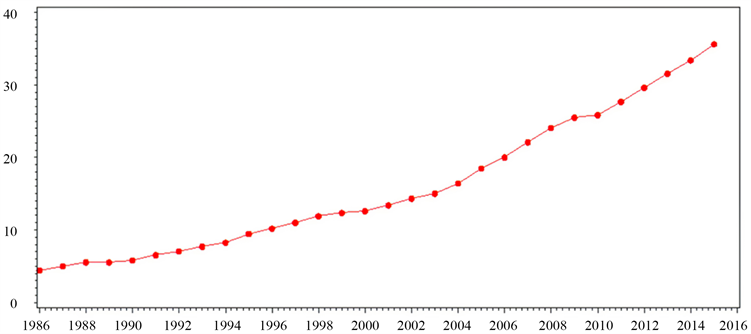Figure 1. Time sequence of total tea production in Fujian Province from 1986 to 2015

Cramer分解定理里说明了任何一个序列的波动都可以视为同时受到了确定性影响和随机影响的综合作用 。平稳序列要求这两方面的影响都是稳定的，而非平稳序列产生的机理则在于它所受到的这两方面的影响至少有一方面是不平稳的。

2.2. 平稳化处理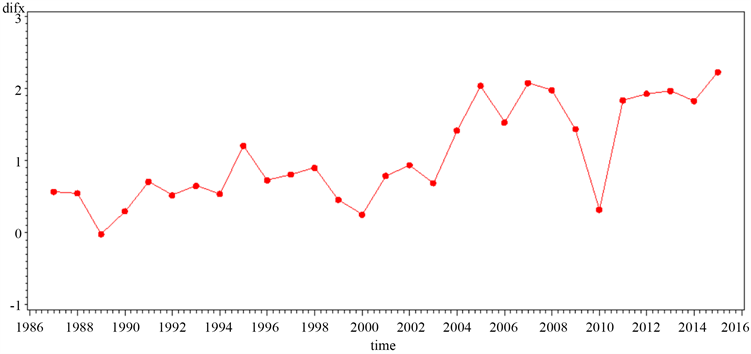Figure 2. Time sequence diagram of the first-order difference of the total output of tea in Fujian Province from 1986 to 2015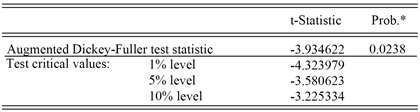Figure 3. Unit root test after first order difference of sequence

2.3. 纯随机性检验

$\text{LB}=n\left(n+2\right){\sum }_{k=1}^{m}\left(\frac{{\stackrel{^}{\rho }}_{k}^{2}}{n-k}\right)$ (1)Table 2. White noise test chart

3. ARIMA(1,1,0)模型识别及参数估计

3.1. 模型识别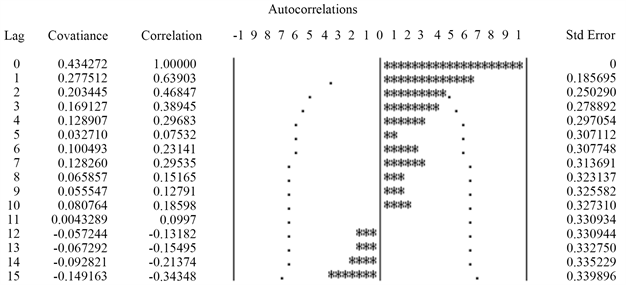Figure 4. Autocorrelation graph after first order difference of sequence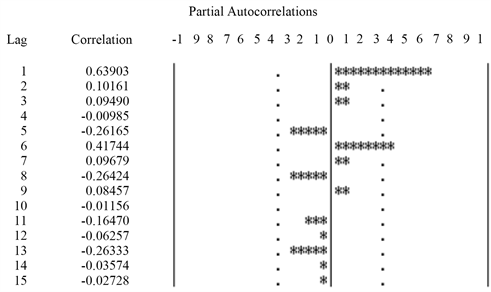Figure 5. Partial autocorrelation graph after first order difference of sequence

3.2. 模型参数估计

$\stackrel{˜}{\beta }={\left({\phi }_{1},\cdots ,{\phi }_{p},{\theta }_{1},\cdots ,{\theta }_{q}\right)}^{\prime }$ (2)

${F}_{t}\left(\stackrel{˜}{\beta }\right)={\phi }_{1}{x}_{t-1}+\cdots +{\phi }_{p}{x}_{t-p}-{\theta }_{1}{\epsilon }_{t-1}-\cdots -{\theta }_{q}{\epsilon }_{t-q}$ (3)

$Q\left(\stackrel{˜}{\beta }\right)={\sum }_{t=1}^{n}{\left[{x}_{t}-{\sum }_{i=1}^{t}{\pi }_{i}{x}_{t-i}\right]}^{2}$ (4)

$\nabla {x}_{t}=1.03544+\frac{{\epsilon }_{t}}{1-0.71939B}$ (5)

${x}_{t}=0.29056+1.71939{x}_{t-1}-0.71939{x}_{t-2}+{\epsilon }_{t}$ (6)

4. ARIMA(1,1,0)模型检验及预测

4.1. 模型检验Table 4. Model significance test tableTable 5. Parameter significance test table

4.2. 模型预测及数据对比Table 6. Prediction table of total output value of tea in Fujian Province in 2016~2019Table 7. Comparison table of total output value of tea in Fujian Province in 2016~2019

5. ARIMA(1,2,1)模型识别及参数估计

5.1. 平稳性检验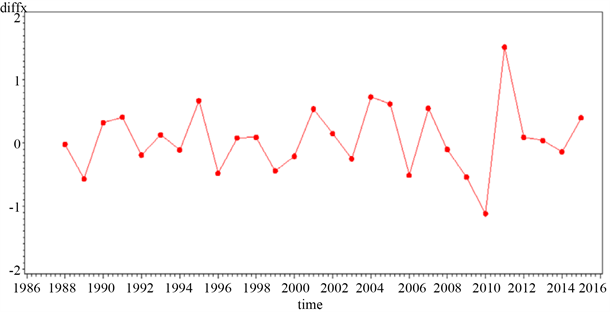Figure 6. Sequence diagram after second order difference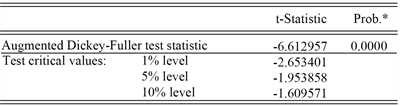Figure 7. Unit root test after second order difference of sequence

5.2. 纯随机性检验Table 8. Second order difference pure random test table

6. ARIMA(1,2,1)模型识别及参数估计

6.1. 模型识别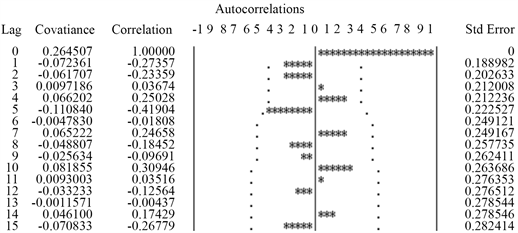Figure 8. Autocorrelation graph after second order difference of sequence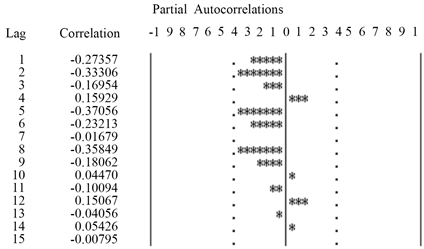Figure 9. Partial autocorrelation graph after second order difference of sequence

6.2. 模型参数估计Table 9. Parameter estimation table after second order difference

${\nabla }^{2}{x}_{t}=0.04228+\frac{1-B}{1-0.47205B}{\epsilon }_{t}$ (7)

7. ARIMA(1,2,1)模型检验及预测Table 10. Significance test table of second-order difference modelTable 11. Test table of parameter significance after second-order differenceTable 12. Parameter significance test table after parameter eliminationTable 13. Parameter estimation table after parameter elimination

${x}_{t}=2{x}_{t-1}-{x}_{t-2}+0.50424{\epsilon }_{t}$ (8)Table 14. Prediction table of total output value of tea in Fujian Province in 2016~2019Table 15. Comparison table of total output value of tea in Fujian Province in 2016~2019

8. 模型对比及预测Table 16. Comparison table of model resultsTable 17. Prediction table of total output value of tea in Fujian Province from 2020 to 2025

9. 结论

 沈德福. 福建三大品牌发展现状与开发路径研究[J]. 福建茶叶, 2019(8): 1-2.

 沈德福. 福建省近二十年茶叶发展态势及地区资源禀赋差异分析[J]. 茶叶, 2019(1): 50-52.

 肖枝洪, 郭明月. 时间序列分析与SAS应用[M]. 武汉: 武汉大学出版社, 2009.

 刘佳, 赵慧文, 刘光荣. 基于SAS的非平稳时间序列分析及实证研究[J]. 汕头大学学报·自然科学, 2010, 25(1): 48-53.

 张亚婕. 基于ARIMA模型对股票和指数预测结果的简单比较分析[J]. 市场研究, 2019(11): 23-25.

 于俊年. 计量经济学软件Eviews的使用[M]. 北京: 对外经济贸易大学出版社, 2012.

 易丹辉, 王燕. 应用时间序列分析[M]. 北京: 中国人民大学出版社, 2019.

 阮敬, 纪宏编. 使用SAS统计分析教程[M]. 北京: 中国统计出版社, 2013.

 郑岩岩. 基于SAS软件的时间序列分析在GDP预测中的应用[J]. 金融经济, 2013(9): 179-181.

Top